Queries for Cores: search statistic / browse statistics / browse maps from / browse maps to

# 1. Definition & Example

• A $k$-core is an integer partition $\lambda$ which has no hooks whose size are multiples of $k$.

• Equivalently, $\lambda$ has no rim hooks that are multiples of $k$ [JK81].

• The length of a $k$-core is the number of boxes in its diagram with hook length less than $k$.

 the 7 Cores of size (5, 6)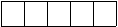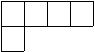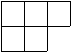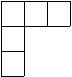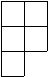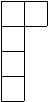(,6) ([4,1],6) ([3,2],6) ([3,1,1],6) ([2,2,1],6) ([2,1,1,1],6) ([1,1,1,1,1],6)
• $k$-cores are graphically represented by the associated Ferrers diagram (or Young diagram) as a collection of boxes.

• For fixed $k \geq 1$, the number $c_k(n)$ of $k$-cores of size $n$ is A175595. The generating function for $c_k(n)$ is $$\sum_{n=0}^\infty c_k(n) q^n = \prod_{n=1}^\infty \frac{(1 - q^{kn})^k}{(1 - q^n)},$$ see [Ono95].

# 2. Properties

• There is a bijective correspondence between $k$-cores and $(k-1)$-bounded partitions. The $(k-1)$-bounded partition corresponding to the $k$-core $\lambda$ is obtained by removing all the cells of $\lambda$ whose hook length is greater than or equal to $k$ [LLM12].

• There is a bijection between $k$-cores and affine Grassmannian elements.

# 3. $(a,b)$-cores

• A partition $\lambda$ is an $(a,b)$-core $\lambda$ if, and only if, it is both an $a$-core and a $b$-core.

• The total number of $(a,b)$-core partitions is finite if, and only if, $a$ and $b$ are coprime, in which case the number is $$\frac{1}{a+b} \binom{a+b}{a,b} = \frac{(a+b-1)!}{a!b!},$$ see [And02].

See [AHJ14] for open questions and conjectures concerning $(a,b)$-cores relating to generalized Catalan numbers and $q,t$-Catalan numbers.

• [AHJ14]   Armstrong, D., Hanusa, C. R. H., Jones, B. C., Results and conjectures on simultaneous core partitions, arXiv:1308.0572 (2014).

[And02]   Anderson, Jaclyn, Partitions which are simultaneously $t_1$- and $t_2$-core, Disc. Math., 248, (2002), pp. 237-243.

[JK81]   James, Gordon; Kerber, Adalbert, The representation theory of the symmetric group, Addison-Wesley Publishing Co., Reading, Mass. (1981).

[LLM12]   Lam, T., Lapointe, L., Morse, J., et al., k-Schur functions and affine Schubert calculus, arXiv:1301.3569 (2012).

[Ono95]   Ono, Ken, A Note on the number of $t$-Core Partitions, Rocky Mountain Journal of Mathematics 25 (1995), no. 3, pp. 1165-1169.

# 6. Technical information for database usage

• A $k$-core is uniquely represented as a pair (X,n) with X being the partition and n its length.

• Cores are graded by pairs (n,k) such that $k$-cores are graded by their lengths.

• The database contains all cores with parameters

$$(2,3),(3,3),(4,3),(5,3),(6,3),(2,4),(3,4),(4,4),(5,4),(6,4),(2,5),(3,5),(4,5),(5,5),(6,5),(2,6),(3,6),(4,6),(5,6),(6,6),(7,6).$$